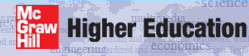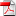Institut für Thermodynamik der Luft- und Raumfahrt - Universität StuttgartMechanical Engineering - The University of Texas at Austinpipe flow

The benchmark dataset for the laminar pipe flow is the classic combined entry flow problem, where the thermal boundary condition is applied at the entrance to the pipe, and both the hydrodynamic and thermal boundary layers develop simultaneously. From a fluid mechanics perspective the flow within the pipe is divided into the hydrodynamic entry length region where the hydrodynamic boundary layer begins developing once the no-slip boundary condition is applied, and the hydrodynamically fully developed flow region that occurs, usually after the hydrodynamic boundary layer development reaches the pipe centerline. From a thermal perspective the flow within the pipe is divided into the thermal entry length region where the thermal boundary layer begins developing once fluid heating or cooling begins, and the thermally fully developed flow region that occurs, usually after the thermal boundary development reaches the pipe centerline. For constant property flow the region far from the entrance will result in the friction coefficient becoming constant (hydrodynamically fully developed) and the heat transfer coefficient becoming constant (thermally fully developed).

Dataset Name - The combined entry length pipe problem is the benchmark dataset s30.dat.txt.

Problem Description - The benchmark laminar pipe flow problem supports CHMT problem 7.9 (for momentum transfer) and 8.27 (for heat transfer). The thermal boundary condition for this problem is a constant surface temperature, designed to heat the fluid, Ts / Tm > 1). The problem description includes:

• ReDh = 1000
• Tm = 300K (the mass-averaged temperature at the inlet)
• Ts = 310K (the surface temperature, constant)
• fluid:
• air at 1 atm (101325 Pa)
• Prandtl number = 0.70 for the thermal analysis
• properties constant
note: this requires no body force or viscous dissipation effects
• geometry:
• circular pipe
• dimensions: 3.5 cm (0.035 m) pipe radius and7.0 m long
• start conditions: at the entrance to the pipe, x = 0.0, using flat initial profiles - note: this is problem 8-22
• end condition: overall length of pipe converts to x+ = (x/Dh)/(ReDhPr) = 0.3 to guarantee computing beyond the thermal entrance length for this Re and for Pr of air

website updated Sept 2010   © 1996-2010 Michael E. Crawford - all rights reserved - website validated for CSS 2.1 and XHTML 1.0 strict at www.w3.org